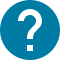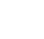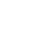学部前期課程
HOME 学部前期課程 数学(PEAK)

# 数学(PEAK)

Single-variable calculus
In this course, we study the differentiation and integration of a function of one-variable (which is also called one-variable function or single-variable function). Intuitively, differential means the slope of the tangent line of a function at a given point, and integral measures the area bounded by the graph of the function and x-axis over a given interval. Both concepts need the concept “limit”, so the first part of this course will contain the definition of a function, limit of a sequence, continuous function and related topics. In the second part of this course, we study the differential of a function, the definition, calculation techniques, properties and applications. In the third part, we define the definite and indefinite integral of a function, study the fundamental theorem of calculus: the connection between differentiation and integration, and introduce the calculation techniques. Power series will also be introduced. In the end, we discuss how to solve some simple differential equations.

コース名

50803
CAS-PF1550L3

A1 A2

2

NO

1. Sequence, limit, continuous function 2. The derivative of a function and higher-order derivatives 3. Taylor's theorem and extreme values 4. Taylor series 5. Integral of a function 6. the fundamental theorem 7. Integration methods 8. Differential equations

Lecture notes and homework will be given each week. I plan to give face-to-face lessons with blackboard, but final decision will be made by peak office.

Final exam+homework. Details will be given during the lecture.

Make sure you are able to spend some time on exercises/homework if you take this course.